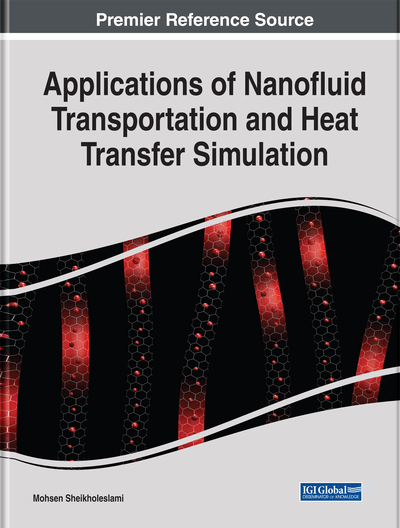# Nanoparticle Transportation in a Porous Medium

DOI: 10.4018/978-1-5225-7595-5.ch005

## Abstract

The study of convective heat transfer in fluid-saturated porous media has many important applications in technology geothermal energy recovery such as oil recovery, food processing, fiber and granular insulation, porous burner and heater, combustion of low-calorific fuels to diesel engines, and design of packed bed reactors. Also, the flow in porous tubes or channels has been under considerable attention in recent years because of its various applications in biomedical engineering, transpiration cooling boundary layer control, and gaseous diffusion. Nanofluids are produced by dispersing the nanometer-scale solid particles into base liquids with low thermal conductivity such as water, ethylene glycol (EG), and oils. In this chapter, nanofluid hydrothermal behavior in porous media has been investigated.
Chapter Preview
Top

## 1. Nanofluid Heat Transfer Over A Permeable Stretching Wall In A Porous Medium

### 1.1. Problem Definition

A steady, constant property, two-dimensional flow of an incompressible nanofluid through a homogenous porous medium with permeability of K, over a stretching surface with linear velocity distribution, i.e.,is assumed (Figure 1) (Sheikholeslami, Ellahi, Ashorynejad, Domairry and Hayat, 2014).

The fluid is a water based nanofluid containing different types of nanoparticles: Cu, Al2O3, Ag and TiO2. It is assumed that the base fluid and the nanoparticles are in thermal equilibrium and no slip occurs between them. The thermo physical properties of the nanofluid are given in Table 1.

The transport properties of the medium can be considered independent from the temperature when the temperature difference between wall and ambient is not significant (Starov and Zhdanov, 2001). The origin is kept fixed while the wall is stretching and the y-axis is perpendicular to the surface. Using the above-mentioned assumptions, the continuity equation is:

(1) where u and v are velocity components in the x and y directions, respectively. The Brinkman model x-momentum equation reads:(2)(3) whereis the effective viscosity which for simplicity in the present study is considered to be identical to the dynamic viscosity,. This assumption is reasonable for packed beds of particles (Hooman, Gurgenci and Merrikh, 2007)

(4)(5)(6)(7)

The hydrodynamic boundary conditions are:

(8) whereis the non-dimensional x-coordinate and L is the length of the porous plate.

The following thermal boundary conditions are considered:

(9)(10)

The power-law temperature and heat flux distribution, described in Equations (9) and (10), resent a wider range of thermal boundary conditions including isoflux and isothermal cases. For example, by setting n equal to zero, Equations (9) and (10) yield isothermal and isoflux, respectively.

Second law of thermodynamics analysis of porous media is found to be more complicated compared to the clear fluid counterpart due to increased number of variables in governing equations (Nield and Kuznetsov, 2005). In the non-Darcian regime, there are three alternative models for the fluid friction term which are the clear-fluid compatible model, the Darcy model, and the Nield model or the power of drag model. Following the entropy generation function introduced by Nield and Kuznetsov (2005) the volumetric entropy generation rate,, reads:

(11)

Using boundary layer approximations, Equation (11) reduces to:

(12)

Using the stream function,, the continuity equation is satisfied:

(13)

The hydrodynamic boundary layer thickness scales with. This can be found through a scale analysis between the first and the second terms on the right hand side of Equation (2), i.e., the viscous and the Darcy terms. Therefore, instead of the other similarity parameters reported in the literature, the following dimensionless similarity parameter is defined

(14)

The u-velocity is assumed to be correlated to, a dimensionless similarity function as:

(15) whereis. Using stream function definition, Equation (15), the stream function and the-velocity take the following forms:

(16)(17) whereis a parameter having the following form:(18) where Re is the Reynolds number. Equation (18) should be solved subjected to the following boundary conditions:

(19)

The wall shear stress is the driving force that drags fluid flow along the stretching wall. The wall shear stress term can then be found, in terms of the similarity function, as:

(20)(21) whereisandfor the power-law temperature and heat flux boundary conditions, respectively. The thermal energy equation reads:(22) whereare parameters having the following form:

(23)

Which are subjected to the following boundary conditions:Power-law temperature(24)Power-law heat flux

For power-law temperature and heat flux boundary conditions, respectively. Employing the definition of convective heat transfer coefficient, the local Nusselt numbers, become:

(25)

Finally, the local volumetric entropy generation rate for the above cases, respectively, reads:

(26) whereis the heat transfer irreversibility due to heat transfer in the direction of finite temperature gradients.is common in all types of thermal engineering applications.

The last term () is the contribution of fluid friction irreversibility to the total entropy generation. Not only the wall and fluid layer shear stress but also the momentum exchange at the solid boundaries (pore level) contributes to. In terms of the primitive variables,andbecome

(27)(28) whereandare measured in degrees of Kelvin.

(29)

The Bejan number shows the ratio of entropy generation due to heat transfer irreversibility to the total entropy generation so that a Be value more/less than 0.5 shows that the contribution ofto the total entropy generation is higher/less than that of. The limiting value ofshows that the only active entropy generation mechanism is HTI whilerepresents nocontribution to the total entropy production.

## Complete Chapter List

Search this Book:
Reset